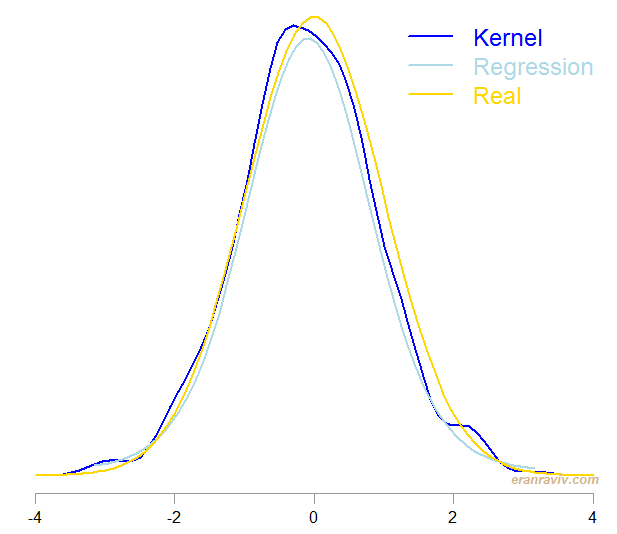# Density Estimation Using Regression

Density estimation using regression? Yes we can!

I like regression. It is one of those simple yet powerful statistical methods. You always know exactly what you are doing. This post is about density estimation, and how to get an estimate of the density using (Poisson) regression.

The “go-to” estimator for density is currently a nonparametric (or semiparametric) kernel. This is the estimator behind the `density` function in R. In the past (references below) there was a line of research directed towards density estimation using regression. Part of the motivation for following this line of research was simplicity and ease of use. I guess it is for the same reason that this line of research dwindled over the years. Increased computational power allows us to do away with simplicity to a certain extent.

There is some sort of law working here whereby statistical methodology always expands to strain the current limits of computation (Bradley Efron). Maximum Likelihood Estimation techniques, which have such strong mathematical, theoretical and asymptotical foundations are now being cornered by more computationally intensive procedures.

Yet I have found this oldish method so nice that I couldn’t resist sharing (so what, you are a geek yourself for reading this).

The idea is as follows (quoted directly from the second reference below):

Estimates of g(y) are traditionally constructed in two quite different ways: by maximum likelihood fitting within some parametric family such as the normal or by nonparametric methods such as kernel density estimation. These two methods can be combined by putting an exponential family ‘through’ a nonparametric estimator.

In simpler words what we can do is use histogram as the nonparametric starting density estimation, and use the counts of each bin (the x) as regressors for the density (the y). This is why Poisson regression is appropriate, the counts are all non-negative integers.

In order to allow for a flexible shape of the density we need to expand the x (the count in each bin) using polynomial expansion. Coding helps clear confusion:

So that is how you can implement the method. You can change the degree of the polynomial so that the density becomes more, or less flexible. Next is a toy example to see how the two methods- the usual go-to kernel estimator and the regression-based estimator, compare on a known density.

I simulated a normal density and estimated it using the two methods, the one which is by Lindsey from 1974 and the usual kernel density, using the `density` function. Here are the results:Both method do quite well. However, the regression-based procedure is easier to follow in my opinion.

## References

• Lindsey, J. K. Comparison of probability distributions. Journal of the Royal Statistical Society. Series B (Methodological) (1974): 38-47.
• Efron, Bradley, and Robert Tibshirani. Using specially designed exponential families for density estimation. The Annals of Statistics 24.6 (1996): 2431-2461.